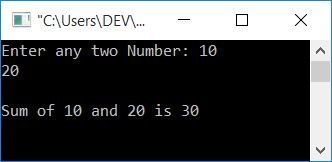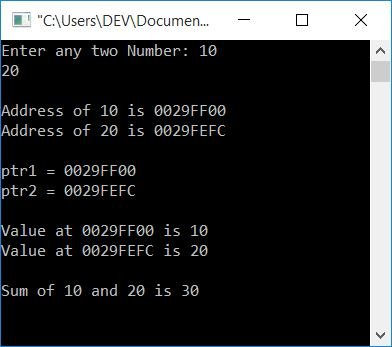# C Program to Add Two Numbers Using a Pointer

In this article, you will learn and get code for adding two numbers in C using a pointer. So the question is, "Write a program in C that adds any two numbers entered by the user (at run-time) using pointers."

## Addition using the pointer in C

To add two numbers using a pointer in C programming, you have to ask the user to enter any two numbers, then perform the operation using a pointer as shown here in the following program. Let's take a look at the program first, and then I will explain it further.

```#include<stdio.h>
#include<conio.h>
int main()
{
int num1, num2, sum;
int *ptr1, *ptr2;
printf("Enter any two Number: ");
scanf("%d%d", &num1, &num2);
ptr1 = &num1;
ptr2 = &num2;
sum = *ptr1 + *ptr2;
printf("\nSum of %d and %d is %d", *ptr1, *ptr2, sum);
getch();
return 0;
}```

This program was written in the Code::Blocks IDE. Here is the initial snapshot of the sample run:Now supply any two numbers as input, say 10 and 20, and press the ENTER key to see the output as shown here in the snapshot given below:In the above program, there are two important operators. The & (ampersand) and * (asterisk) operators. The & represents the operator's address, and the * represents the operator's value.

#### Program Explained

• Declare three variables, say num1, num2,, and sum of int (integer).
• Here, the first two variables stores the two numbers entered by the user at run-time.
• And the third variable, sum, will be used to store the summation of two given numbers using pointers.
• Declare two variables. Assume ptr1 and ptr2 of int pointer (integer pointers).
• To declare a variable as a pointer type variable, simply place an asterisk (*) before it without including any spaces.
• At runtime, scan two numbers. That is, the user is asked to enter any two numbers and store them in the num1 and num2 variables.
• Initialize the address of the first variable that holds the first number, num1, to ptr1 using the & (address of) operator.
• Initialize the address of the second variable that holds the second number, num2, to ptr2 using the & (address of) operator.
• Perform the operation.
value at ptr1 + value at ptr2
using the * (value at address) operator. That is,
`*ptr1 + *ptr2`
As both the variables: ptr1 and ptr2 holds the address of the two given numbers. Finally, initialize it to the variable sum, which holds the sum of the two numbers supplied by the user.
• As output, print the sum value.

Using the address of the (&) operator, we initialized the address of the first number to the first pointer and the address of the second number to the second pointer. And then, we have added the two numbers using the value at address (*) operator and initialized it to the variable sum. Finally, as an addition result, I printed the sum value.

Here is another program that provides you with a full understanding of pointers after watching its output carefully. Let's take a look at the program:

```#include<stdio.h>
#include<conio.h>
int main()
{
int num1, num2, sum;
int *ptr1, *ptr2;
printf("Enter any two Number: ");
scanf("%d%d", &num1, &num2);
printf("\nAddress of %d is %p", num1, &num1);
printf("\nAddress of %d is %p", num2, &num2);
ptr1 = &num1;
ptr2 = &num2;
printf("\n\nptr1 = %p", ptr1);
printf("\nptr2 = %p", ptr2);
printf("\n\nValue at %p is %d", ptr1, *ptr1);
printf("\nValue at %p is %d", ptr2, *ptr2);
sum = *ptr1 + *ptr2;
printf("\n\nSum of %d and %d is %d", *ptr1, *ptr2, sum);
getch();
return 0;
}```

The output of the above program is shown in the snapshot given below:The format specifier %p is used for the standard notation of memory's address.

#### The same program in different languages

C Quiz

« Previous Program Next Program »